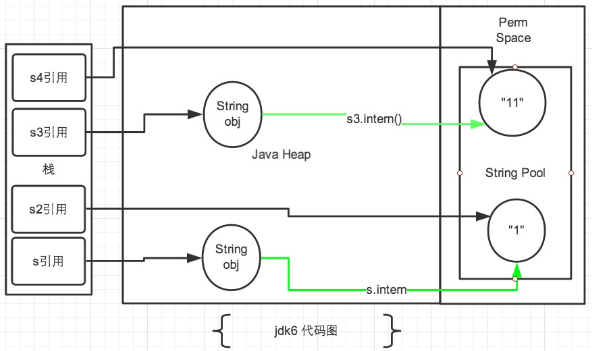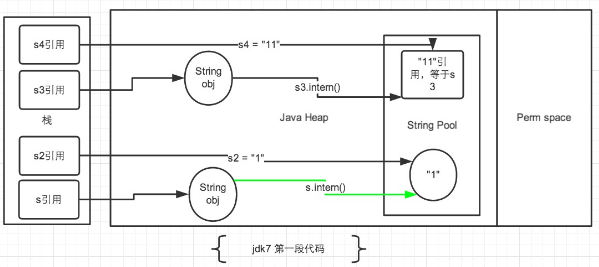# Java 基本数据类型## Java 的两大数据类型:

• 内置数据类型

• 引用数据类型

### 内置数据类型

Java语言提供了八种基本类型。六种数字类型四个整数型两个浮点型一种字符类型还有一种布尔型。

byte

• byte 数据类型是8位、有符号的以二进制补码表示的整数

• 最小值是 -128-2^7

• 最大值是 1272^7-1

• 默认值是 0

• byte 类型用在大型数组中节约空间主要代替整数因为 byte 变量占用的空间只有 int 类型的四分之一

• 例子byte a = 100byte b = -50。

short

• short 数据类型是 16 位、有符号的以二进制补码表示的整数

• 最小值是 -32768-2^15

• 最大值是 327672^15 - 1

• Short 数据类型也可以像 byte 那样节省空间。一个short变量是int型变量所占空间的二分之一

• 默认值是 0

• 例子short s = 1000short r = -20000。

int

• int 数据类型是32位、有符号的以二进制补码表示的整数

• 最小值是 -2,147,483,648-2^31

• 最大值是 2,147,483,6472^31 - 1

• 一般地整型变量默认为 int 类型

• 默认值是 0

• 例子int a = 100000, int b = -200000。

long

• long 数据类型是 64 位、有符号的以二进制补码表示的整数

• 最小值是 -9,223,372,036,854,775,808-2^63

• 最大值是 9,223,372,036,854,775,8072^63 -1

• 这种类型主要使用在需要比较大整数的系统上

• 默认值是 0L

• 例子 long a = 100000LLong b = -200000L。 "L"理论上不分大小写但是若写成"l"容易与数字"1"混淆不容易分辩。所以最好大写。

float

• float 数据类型是单精度、32位、符合IEEE 754标准的浮点数

• float 在储存大型浮点数组的时候可节省内存空间

• 默认值是 0.0f

• 浮点数不能用来表示精确的值如货币

• 例子float f1 = 234.5f。

double

• double 数据类型是双精度、64 位、符合IEEE 754标准的浮点数

• 浮点数的默认类型为double类型

• double类型同样不能表示精确的值如货币

• 默认值是 0.0d

• 例子double d1 = 123.4。

boolean

• boolean数据类型表示一位的信息

• 只有两个取值true 和 false

• 这种类型只作为一种标志来记录 true/false 情况

• 默认值是 false

• 例子boolean one = true。

char

• char类型是一个单一的 16 位 Unicode 字符

• 最小值是 \u0000即为0

• 最大值是 \uffff即为65,535

• char 数据类型可以储存任何字符

• 例子char letter = ‘A’;。

//8位
byte bx = Byte.MAX_VALUE;
byte bn = Byte.MIN_VALUE;
//16位
short sx = Short.MAX_VALUE;
short sn = Short.MIN_VALUE;
//32位
int ix = Integer.MAX_VALUE;
int in = Integer.MIN_VALUE;
//64位
long lx = Long.MAX_VALUE;
long ln = Long.MIN_VALUE;
//32位
float fx = Float.MAX_VALUE;
float fn = Float.MIN_VALUE;
//64位
double dx = Double.MAX_VALUE;
double dn = Double.MIN_VALUE;
//16位
char cx = Character.MAX_VALUE;
char cn = Character.MIN_VALUE;
//1位
boolean bt = Boolean.TRUE;
boolean bf = Boolean.FALSE;

`127` `-128` `32767` `-32768` `2147483647` `-2147483648` `9223372036854775807` `-9223372036854775808` `3.4028235E38` `1.4E-45` `1.7976931348623157E308` `4.9E-324`

`true` `false`

### 引用类型

• 在Java中引用类型的变量非常类似于C/C++的指针。引用类型指向一个对象指向对象的变量是引用变量。这些变量在声明时被指定为一个特定的类型比如 Employee、Puppy 等。变量一旦声明后类型就不能被改变了。

• 对象、数组都是引用数据类型。

• 所有引用类型的默认值都是null。

• 一个引用变量可以用来引用任何与之兼容的类型。

• 例子Site site = new Site(“Runoob”)。

### Java 常量

final double PI = 3.1415927;

byte a = 68;
char a = ‘A’

## 自动拆箱和装箱详解

Java 5增加了自动装箱与自动拆箱机制方便基本类型与包装类型的相互转换操作。在Java 5之前如果要将一个int型的值转换成对应的包装器类型Integer必须显式的使用new创建一个新的Integer对象或者调用静态方法Integer.valueOf()。

//在Java 5之前只能这样做 Integer value = new Integer(10); //或者这样做 Integer value = Integer.valueOf(10); //直接赋值是错误的 //Integer value = 10;`

`//在Java 5中直接赋值是合法的由编译器来完成转换` `Integer value = 10;` `与此对应的自动拆箱就是可以将包装类型转换为基本类型具体的转换工作由编译器来完成。` `//在Java 5 中可以直接这么做` `Integer value = new Integer(10);` `int i = value;`

### 简易实现

class Auto //code1 { public static void main(String[] args) { //自动装箱 Integer inte = 10; //自动拆箱 int i = inte;

//再double和Double来验证一下
Double doub = 12.40;
double d = doub;

}

} 上面的代码先将int型转为Integer对象再讲Integer对象转换为int型毫无疑问这是可以正确运行的。可是这种转换是怎么进行的呢使用反编译工具将生成的Class文件在反编译为Java文件让我们看看发生了什么 class Auto//code2 { public static void main(String[] paramArrayOfString) { Integer localInteger = Integer.valueOf(10);

int i = localInteger.intValue();

Double localDouble = Double.valueOf(12.4D);
double d = localDouble.doubleValue();

} } 我们可以看到经过javac编译之后code1的代码被转换成了code2实际运行时虚拟机运行的就是code2的代码。也就是说虚拟机根本不知道有自动拆箱和自动装箱这回事在将Java源文件编译为class文件的过程中javac编译器在自动装箱的时候调用了Integer.valueOf()方法在自动拆箱时又调用了intValue()方法。我们可以看到double和Double也是如此。 实现总结其实自动装箱和自动封箱是编译器为我们提供的一颗语法糖。在自动装箱时编译器调用包装类型的valueOf()方法在自动拆箱时编译器调用了相应的xxxValue()方法。

### 自动装箱与拆箱中的“坑”

Integer源码

public final class Integer extends Number implements Comparable { private final int value;

/Integer的构造方法接受一个整型参数,Integer对象表示的int值保存在value中/
public Integer(int value) {
this.value = value;
}

/equals()方法判断的是:所代表的int型的值是否相等/
public boolean equals(Object obj) {
if (obj instanceof Integer) {
return value == ((Integer)obj).intValue();
}
return false;
}

/返回这个Integer对象代表的int值也就是保存在value中的值/
public int intValue() {
return value;
}

/**

• 首先会判断i是否在[IntegerCache.low,Integer.high]之间
• 如果是直接返回Integer.cache中相应的元素
• 否则调用构造方法创建一个新的Integer对象
/
public static Integer valueOf(int i) {
assert IntegerCache.high >= 127;
if (i >= IntegerCache.low && i <= IntegerCache.high)
return IntegerCache.cache[i + (-IntegerCache.low)];
return new Integer(i);
}

/
*
• 静态内部类缓存了从[low,high]对应的Integer对象
• low -128这个值不会被改变
• high 默认是127可以改变最大不超过Integer.MAX_VALUE - (-low) -1
• cache 保存从[low,high]对象的Integer对象
*/
private static class IntegerCache {
static final int low = -128;
static final int high;
static final Integer cache[];

static {
// high value may be configured by property
int h = 127;
String integerCacheHighPropValue =
sun.misc.VM.getSavedProperty(“java.lang.Integer.IntegerCache.high”);
if (integerCacheHighPropValue != null) {
int i = parseInt(integerCacheHighPropValue);
i = Math.max(i, 127);
// Maximum array size is Integer.MAX_VALUE
h = Math.min(i, Integer.MAX_VALUE - (-low) -1);
}
high = h;

cache = new Integer[(high - low) + 1];
int j = low;
for(int k = 0; k < cache.length; k++)
cache[k] = new Integer(j++);
}

private IntegerCache() {}
}

} 以上是Oracle(Sun)公司JDK 1.7中Integer源码的一部分通过分析上面的代码得到 1Integer有一个实例域value它保存了这个Integer所代表的int型的值且它是final的也就是说这个Integer对象一经构造完成它所代表的值就不能再被改变。 2Integer重写了equals()方法它通过比较两个Integer对象的value来判断是否相等。 3重点是静态内部类IntegerCache通过类名就可以发现它是用来缓存数据的。它有一个数组里面保存的是连续的Integer对象。 (a) low代表缓存数据中最小的值固定是-128。 (b) high代表缓存数据中最大的值它可以被该改变默认是127。high最小是127最大是Integer.MAX_VALUE-(-low)-1如果high超过了这个值那么cache[ ]的长度就超过Integer.MAX_VALUE了也就溢出了。 © cache[]里面保存着从[low,high]所对应的Integer对象长度是high-low+1(因为有元素0所以要加1)。 4调用valueOf(int i)方法时首先判断i是否在[low,high]之间如果是则复用Integer.cache[i-low]。比如如果Integer.valueOf(3)直接返回Integer.cache如果i不在这个范围则调用构造方法构造出一个新的Integer对象。 5调用intValue()直接返回value的值。 通过3和4可以发现默认情况下在使用自动装箱时VM会复用[-128,127]之间的Integer对象。

Integer a1 = 1; Integer a2 = 1; Integer a3 = new Integer(1); //会打印true因为a1和a2是同一个对象,都是Integer.cache System.out.println(a1 == a2); //falsea3构造了一个新的对象不同于a1,a2 System.out.println(a1 == a3);

### 了解基本类型缓存常量池的最佳实践

//基本数据类型的常量池是-128到127之间。
// 在这个范围中的基本数据类的包装类可以自动拆箱比较时直接比较数值大小。
public static void main(String[] args) {
//int的自动拆箱和装箱只在-128到127范围中进行超过该范围的两个integer的 == 判断是会返回false的。
Integer a1 = 128;
Integer a2 = -128;
Integer a3 = -128;
Integer a4 = 128;
System.out.println(a1 == a4);
System.out.println(a2 == a3);

Byte b1 = 127;
Byte b2 = 127;
Byte b3 = -128;
Byte b4 = -128;
//byte都是相等的因为范围就在-128到127之间
System.out.println(b1 == b2);
System.out.println(b3 == b4);

//
Long c1 = 128L;
Long c2 = 128L;
Long c3 = -128L;
Long c4 = -128L;
System.out.println(c1 == c2);
System.out.println(c3 == c4);

//char没有负值
//发现char也是在0到127之间自动拆箱
Character d1 = 128;
Character d2 = 128;
Character d3 = 127;
Character d4 = 127;
System.out.println(d1 == d2);
System.out.println(d3 == d4);

`结果`

`false` `true` `true` `true` `false` `true` `false` `true`

Integer i = 10;
Byte b = 10;
//比较Byte和Integer.两个对象无法直接比较报错
//System.out.println(i == b);
System.out.println("i == b " + i.equals(b));
//答案是false,因为包装类的比较时先比较是否是同一个类不是的话直接返回false.
int ii = 128;
short ss = 128;
long ll = 128;
char cc = 128;
System.out.println("ii == bb " + (ii == ss));
System.out.println("ii == ll " + (ii == ll));
System.out.println("ii == cc " + (ii == cc));

i == b false
ii == bb true
ii == ll true
ii == cc true

//这时候都是true因为基本数据类型直接比较值值一样就可以。

### 总结

1当需要一个对象的时候会自动装箱比如Integer a = 10;equals(Object o)方法的参数是Object对象所以需要装箱。

2当需要一个基本类型时会自动拆箱比如int a = new Integer(10);算术运算是在基本类型间进行的所以当遇到算术运算时会自动拆箱比如代码中的 c == (a + b);

3 包装类型 == 基本类型时包装类型自动拆箱

1Integer默认复用了[-128,127]这些对象其中高位置可以修改

2Byte复用了全部256个对象[-128,127]

3Short服用了[-128,127]这些对象

4Long服用了[-128,127];

5Character复用了[0,127],Charater不能表示负数;

Double和Float是连续不可数的所以没法复用对象也就不存在自动装箱复用陷阱。

Boolean没有自动装箱与拆箱它也复用了Boolean.TRUE和Boolean.FALSE通过Boolean.valueOf(boolean b)返回的Blooean对象要么是TRUE要么是FALSE这点也要注意。

## 基本数据类型的存储方式

### 存在栈中

public void(int a) { int i = 1; int j = 1; } 方法中的i 存在虚拟机栈的局部变量表里i是一个引用j也是一个引用它们都指向局部变量表里的整型值 1. int a是传值引用所以a也会存在局部变量表。

### 存在堆里

class A{ int i = 1; A a = new A(); } i是类的成员变量。类实例化的对象存在堆中所以成员变量也存在堆中引用a存的是对象的地址引用i存的是值这个值1也会存在堆中。可以理解为引用i指向了这个值1。也可以理解为i就是1.

3 包装类对象怎么存 其实我们说的常量池也可以叫对象池。 比如String a= new String(“a”).intern()时会先在常量池找是否有“a"对象如果有的话直接返回“a"对象在常量池的地址即让引用a指向常量”a"对象的内存地址。 public native String intern(); Integer也是同理。

public static Integer valueOf(int i) {
if (i >= IntegerCache.low && i <= IntegerCache.high)
return IntegerCache.cache[i + (-IntegerCache.low)];
return new Integer(i);
}
private static class IntegerCache {
static final int low = -128;
static final int high;
static final Integer cache[];

static {
// high value may be configured by property
int h = 127;
String integerCacheHighPropValue =
sun.misc.VM.getSavedProperty(“java.lang.Integer.IntegerCache.high”);
if (integerCacheHighPropValue != null) {
try {
int i = parseInt(integerCacheHighPropValue);
i = Math.max(i, 127);
// Maximum array size is Integer.MAX_VALUE
h = Math.min(i, Integer.MAX_VALUE - (-low) -1);
} catch( NumberFormatException nfe) {
// If the property cannot be parsed into an int, ignore it.
}
}
high = h;

cache = new Integer[(high - low) + 1];
int j = low;
for(int k = 0; k < cache.length; k++)
cache[k] = new Integer(j++);

// range [-128, 127] must be interned (JLS7 5.1.7)
assert IntegerCache.high >= 127;
}

private IntegerCache() {}
}

JDK1.7后常量池被放入到堆空间中这导致intern()函数的功能不同具体怎么个不同法且看看下面代码这个例子是网上流传较广的一个例子分析图也是直接粘贴过来的这里我会用自己的理解去解释这个例子

[java] view plain copy
String s = new String(“1”);
s.intern();
String s2 = “1”;
System.out.println(s == s2);

String s3 = new String(“1”) + new String(“1”);
s3.intern();
String s4 = “11”;
System.out.println(s3 == s4);

[java] view plain copy
JDK1.6以及以下false false
JDK1.7以及以上false trueJDK1.6查找到常量池存在相同值的对象时会直接返回该对象的地址。

JDK 1.7后intern方法还是会先去查询常量池中是否有已经存在如果存在则返回常量池中的引用这一点与之前没有区别区别在于如果在常量池找不到对应的字符串则不会再将字符串拷贝到常量池而只是在常量池中生成一个对原字符串的引用。

## 参考文章

0人推荐随时随地看视频慕课网APP